• 基于C++的虹膜识别测试工程为：基于C++和Opencv2的虹膜识别工程。基于Python的测试代码为：https://github.com/thuyngch/Iris-Recognition。 二、测试处理 为了使用相同的数据集进行测试，本文以Python版本的虹膜...
目录

一、说明

二、测试处理

1、基于Python的虹膜识别

2、基于C++虹膜识别

三、测试结果

一、说明

本文主要对基于C++和Python的虹膜识别的效果进行测试，并对比其结果。基于C++的虹膜识别测试工程为：基于C++和Opencv2的虹膜识别工程。基于Python的测试代码为：https://github.com/thuyngch/Iris-Recognition。

二、测试处理

为了使用相同的数据集进行测试，本文以Python版本的虹膜识别为主，保存测试过程中使用的测试图片，然后用C++版本对这些图片进行测试，测试结果的评价标准也按照Python版本中的标准：

fscore = 2*precision*recall / (precision+recall)

1、基于Python的虹膜识别

主要以代码https://github.com/thuyngch/Iris-Recognition为主，使用说明在原代码中已经很详细，本文使用的环境为：Window10、Python3.7.3。需要注意的几点如下：

（1）虹膜识别的测试文件为：eval_casia1.py，需要到python文件加下运行：python3 eval_casia1.py。注意代码中测试数据集CASIA-IrisV1存放路径：

CASIA1_DIR = "../CASIA1"

（2）直接运行python3 eval_casia1.py会出错，根据错误提示，添加了

if __name__ == '__main__':

即代码为：

if __name__ == '__main__':
#------------------------------------------------------------------------------
#	Main execution
#------------------------------------------------------------------------------
# Get identities of MMU2 dataset
identities = glob(os.path.join(CASIA1_DIR, "**"))
identities = sorted([os.path.basename(identity) for identity in identities])
n_identities = len(identities)
print("Number of identities:", n_identities)

# Construct a dictionary of files
files_dict = {}
image_files = []
for identity in identities:
files = glob(os.path.join(CASIA1_DIR, identity, "*.*"))
shuffle(files)
files_dict[identity] = files[:N_IMAGES]
image_files += files[:N_IMAGES]

n_image_files = len(image_files)
print("Number of image files:", n_image_files)
# save test images to file 'pro_imgs.txt'
txt_open = open('./pro_imgs.txt', 'w')
for i in image_files:
line = i + '\n'
txt_open.write(line)
txt_open.close()

# Ground truth
ground_truth = np.zeros([n_image_files, n_image_files], dtype=int)
for i in range(ground_truth.shape):
for j in range(ground_truth.shape):
if i//N_IMAGES == j//N_IMAGES:
ground_truth[i, j] = 1
# Evaluate parameters
pools = Pool(processes=cpu_count())
best_results = []
for eye_threshold in tqdm(eyelashes_thresholds, total=len(eyelashes_thresholds)):
# Extract features
args = zip(image_files, repeat(eye_threshold), repeat(False))
features = list(pools.map(pool_func_extract_feature, args))

# Calculate the distances
args = []
for i in range(n_image_files):
for j in range(n_image_files):
if i>=j:
continue
arg = (features[i], features[i], features[j], features[j])
args.append(arg)
distances = pools.map(pool_func_calHammingDist, args)

# Construct a distance matrix
k = 0
dist_mat = np.zeros([n_image_files, n_image_files])
for i in range(n_image_files):
for j in range(n_image_files):
if i<j:
dist_mat[i, j] = distances[k]
k += 1
elif i>j:
dist_mat[i, j] = dist_mat[j, i]

# Metrics
accuracies, precisions, recalls, fscores = [], [], [], []
for threshold in thresholds:
decision_map = (dist_mat<=threshold).astype(int)
accuracy = (decision_map==ground_truth).sum() / ground_truth.size
precision = (ground_truth*decision_map).sum() / decision_map.sum()
recall = (ground_truth*decision_map).sum() / ground_truth.sum()
fscore = 2*precision*recall / (precision+recall)
accuracies.append(accuracy)
precisions.append(precision)
recalls.append(recall)
fscores.append(fscore)

# Save the best result
best_fscore = max(fscores)
best_threshold = thresholds[fscores.index(best_fscore)]
best_accuracy = accuracies[fscores.index(best_fscore)]
best_precision = precisions[fscores.index(best_fscore)]
best_recall = recalls[fscores.index(best_fscore)]
best_results.append((eye_threshold, best_threshold, best_fscore, best_accuracy, best_precision, best_recall))

# Show the final best result
eye_thresholds = [item for item in best_results]
thresholds = [item for item in best_results]
fscores = [item for item in best_results]
accuracies = [item for item in best_results]
precisions = [item for item in best_results]
recalls = [item for item in best_results]

print("Maximum fscore: ", max(fscores))
print("Best accuracy: ", accuracies[fscores.index(max(fscores))])
print("Best precision: ", precisions[fscores.index(max(fscores))])
print("Best recall: ", recalls[fscores.index(max(fscores))])
print("Best eye_threshold: ", eye_thresholds[fscores.index(max(fscores))])
print("Best threshold: ", thresholds[fscores.index(max(fscores))])

CASIA-IrisV1数据集共有108人，每人为7张虹膜图片，代码中随机从7张图片中选取4个：


N_IMAGES = 4

故总测试图片数为：

108*4=432

将随机选取的图片进行保存：

 # save test images to file 'pro_imgs.txt'
txt_open = open('./pro_imgs.txt', 'w')
for i in image_files:
line = i + '\n'
txt_open.write(line)
txt_open.close()

代码中有两个参数：eyelashes_thresholds和thresholds。eyelashes_thresholds为眼睫毛的阈值，范围为[10，250]，选取25个数值：

eyelashes_thresholds = np.linspace(start=10, stop=250, num=25)

[ 10. 20. 30. 40. 50. 60. 70. 80. 90. 100. 110. 120. 130. 140. 150. 160. 170. 180. 190. 200. 210. 220. 230. 240. 250.]

thresholds为判断两个虹膜是否为同一个的阈值，两个虹膜的差异值小于thresholds则为同一人，否则为不同人。取值范围为[0, 1]，选取100个，步长为:

（1-0）/（100-1）= 0.01010101

thresholds = np.linspace(start=0.0, stop=1.0, num=100)

[0. 0.01010101 0.02020202 0.03030303 0.04040404 0.05050505 0.06060606 0.07070707 0.08080808 0.09090909 0.1010101 0.11111111 0.12121212 0.13131313 0.14141414 0.15151515 0.16161616 0.17171717 0.18181818 0.19191919 0.2020202 0.21212121 0.22222222 0.23232323 0.24242424 0.25252525 0.26262626 0.27272727 0.28282828 0.29292929 0.3030303 0.31313131 0.32323232 0.33333333 0.34343434 0.35353535 0.36363636 0.37373737 0.38383838 0.39393939 0.4040404 0.41414141 0.42424242 0.43434343 0.44444444 0.45454545 0.46464646 0.47474747 0.48484848 0.49494949 0.50505051 0.51515152 0.52525253 0.53535354 0.54545455 0.55555556 0.56565657 0.57575758 0.58585859 0.5959596 0.60606061 0.61616162 0.62626263 0.63636364 0.64646465 0.65656566 0.66666667 0.67676768 0.68686869 0.6969697 0.70707071 0.71717172 0.72727273 0.73737374 0.74747475 0.75757576 0.76767677 0.77777778 0.78787879 0.7979798 0.80808081 0.81818182 0.82828283 0.83838384 0.84848485 0.85858586 0.86868687 0.87878788 0.88888889 0.8989899 0.90909091 0.91919192 0.92929293 0.93939394 0.94949495 0.95959596 0.96969697 0.97979798 0.98989899 1. ]

（3）注意运行路径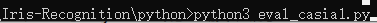2、基于C++虹膜识别

具体代码详见：基于C++和Opencv2的虹膜识别工程。原代码中测试图片为：下一个与上一个进行比较。为了和Python代码保持一致，需要做下修改。

（1）将CASIA1测试数据图片复制到data文件夹中；

（2）修改process.ini文件。修改图片存放文件名称为Python中保存的文件：

Load List of images = pro_imgs.txt

修改图片存放路径：

屏蔽所有保存路径：

#Save segmented images = Output/SegmentedImages/

#Save contours parameters = Output/CircleParameters/

#Save normalized images = Output/NormalizedImages/

#Save iris codes = Output/IrisCodes/

#Save matching scores = Output/score.txt

（3）添加my_run()。在OsiManager.h中加入void my_run()；如下图所示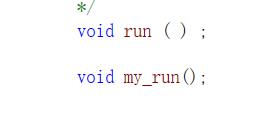在OsiManager.cpp中添加：

void OsiManager::my_run()
{
cout << endl;
cout << "================" << endl;
cout << "Start processing" << endl;
cout << "================" << endl;
cout << endl;

// If matching is requested, create a file
ofstream result_matching;
if (mProcessMatching && mOutputFileMatchingScores != "")
{
try
{
result_matching.open(mOutputFileMatchingScores.c_str(), ios::out);
}
catch (exception & e)
{
cout << e.what() << endl;
throw runtime_error("Cannot create the file for matching scores : " + mOutputFileMatchingScores);
}
}

int num_test_imgs = mListOfImages.size();
int N_IMAGES = 4;
float threshold = 0.393939393939394;
int decision_map_num = 0;
int ground_truth_num = 0;
// ground_truth and dist_mat initial
bool(*ground_truth) = new bool[num_test_imgs];
float(*dist_mat) = new float[num_test_imgs];
bool(*decision_map) = new bool[num_test_imgs];
for (int i = 0; i<num_test_imgs; ++i)
{
for (int j = 0; j<num_test_imgs; ++j)
{
if (i / N_IMAGES == j / N_IMAGES)
{
ground_truth[i][j] = 1;
ground_truth_num++;
}
else
ground_truth[i][j] = 0;

dist_mat[i][j] = 0;
decision_map[i][j] = 0;
}
}
//end ground_truth and dist_mat initial
vector<OsiEye> eyr_res;
cout << "Extract Features start!" << endl;
for (int i = 0; i < num_test_imgs; i++)
{
OsiEye eye;
processOneEye(mListOfImages[i], eye);
eyr_res.push_back(eye);
cout << i + 1 << " pic is over!!!" << endl;
}
cout << "Extract Features end!!!" << endl;

for (int i = 0; i < num_test_imgs; i++)
{
for (int j = 0; j < num_test_imgs; ++j)
{
cout << "start: " << i << " " << j << " ";
if (i < j)
{
OsiEye eyr_i = eyr_res[i];
OsiEye eyr_j = eyr_res[j];
float score = (eyr_res[i]).match((eyr_res[j]), mpApplicationPoints);
dist_mat[i][j] = score;
}
else if (i > j)
{
dist_mat[i][j] = dist_mat[j][i];
}

if (dist_mat[i][j] < threshold)
{
decision_map[i][j] = 1;
decision_map_num++;
}

cout << dist_mat[i][j] << " " << decision_map[i][j] << endl;
}
}
// result
int accuracy_num = 0;
int precision_num = 0;
for (int i = 0; i < num_test_imgs; i++)
{
for (int j = 0; j < num_test_imgs; ++j)
{
if (decision_map[i][j] == ground_truth[i][j])
{
accuracy_num++;
}

if (decision_map[i][j] && ground_truth[i][j])
{
precision_num++;
}
}
}

float accuracy = float(accuracy_num) / float(num_test_imgs*num_test_imgs);
float precision_res = float(precision_num) / float(decision_map_num);
float recall = float(precision_num) / float(ground_truth_num);
float fscore = 2 * precision_res*recall / (precision_res + recall);
cout << "Result: " << endl << "accuracy: " << accuracy << "\t"
<< "precision_res: " << precision_res << "\t"
<< "recall: " << recall << "\t"
<< "fscore: " << fscore << endl;
// end result

//将阈值从0到1按照0.01步长递增，寻找最优的fscore值，并获取对应的最优阈值
float fscore_best = 0.0;
float threshold_best = 0.0;
float recall_best = 0.0;
float precision_best = 0.0;
float accuracy_best = 0.0;
for (threshold = 0.0; threshold < 1.0; threshold += 0.01010101)
{
int accuracy_num = 0;
int precision_num = 0;
decision_map_num = 0;
for (int i = 0; i < num_test_imgs; ++i)
{
for (int j = 0; j < num_test_imgs; ++j)
{
if (dist_mat[i][j] < threshold)
{
decision_map[i][j] = 1;
decision_map_num++;
}

if (decision_map[i][j] == ground_truth[i][j])
{
accuracy_num++;
}

if (decision_map[i][j] && ground_truth[i][j])
{
precision_num++;
}
}
}
float accuracy = float(accuracy_num) / float(num_test_imgs*num_test_imgs);
float precision_res = float(precision_num) / float(decision_map_num);
float recall = float(precision_num) / float(ground_truth_num);
float fscore = 2 * precision_res*recall / (precision_res + recall);
cout << "Result:   " << "accuracy: " << accuracy << "\t"
<< "precision_res: " << precision_res << "\t"
<< "recall: " << recall << "\t"
<< "fscore: " << fscore << endl;

if (fscore > fscore_best)
{
fscore_best = fscore;
threshold_best = threshold;
recall_best = recall;
precision_best = precision_res;
accuracy_best = accuracy;
}
for (int i = 0; i<num_test_imgs; ++i)
{
for (int j = 0; j<num_test_imgs; ++j)
{
decision_map[i][j] = 0;
}
}
}
cout << "Best fscore is: " << fscore_best << ", Best threshold is: " << threshold_best <<
", Best recall is: " << recall_best << ", Best precision is: " << precision_best << ", Best accuracy is: " << accuracy_best << endl;

// save dist_mat
ofstream result_dist;
string result_dist_path = "../data/dist_mat.txt";
try
{
result_dist.open(result_dist_path.c_str(), ios::out);
}
catch (exception & e)
{
cout << e.what() << endl;
throw runtime_error("Cannot create the file for result_dist_path scores : " + result_dist_path);
}

if (result_dist)
{
try
{
for (int i = 0; i < num_test_imgs; i++)
{
int j = 0;
for (j = 0; j < num_test_imgs - 1; ++j)
{
result_dist << dist_mat[i][j] << " ";
}
result_dist << dist_mat[i][j] << "\n";
}
}
catch (exception & e)
{
cout << e.what() << endl;
throw runtime_error("Error while saving result of matching in " + mOutputFileMatchingScores);
}
}
// end save dist_mat

cout << endl;
cout << "==============" << endl;
cout << "End processing" << endl;
cout << "==============" << endl;
cout << endl;

} // end of function

注意：这里只选取了Python代码中的thresholds作为最优结果选择参数，其他参数使用原C++代码中默认的。

（4）若在上述代码中保存了decision_map.txt文件，则寻找最优阈值的代码如下：

void test_best_thr(string test_resuilt_file)
{
// Open the file
ifstream file(test_resuilt_file.c_str(), ifstream::in);

if (!file.good())
throw runtime_error("Cannot read configuration file " + test_resuilt_file);

int decision_map_num = 0;
int ground_truth_num = 0;
int N_IMAGES = 4;
int num_test_imgs = 432; //测试图片总数，定义选取N_IMAGES时，总数为：N_IMAGES*108（108为数据集CASIA1中人的数量，即文件夹的数量）

// ground_truth and dist_mat initial
bool(*ground_truth) = new bool[num_test_imgs]; //定义ground_truth变量
float(*dist_mat) = new float[num_test_imgs];  //定义测试结果dist_mat变量
bool(*decision_map) = new bool[num_test_imgs]; // 根据测试结果和设置的阈值得到的正确与否（0/1）的变量
// 变量初始化
for (int i = 0; i<num_test_imgs; ++i)
{
for (int j = 0; j<num_test_imgs; ++j)
{
if (i / N_IMAGES == j / N_IMAGES)
{
ground_truth[i][j] = 1;
ground_truth_num++;
}
else
ground_truth[i][j] = 0;

dist_mat[i][j] = 0;
decision_map[i][j] = 0;
}
}

// 读取结果文件并按照结果文件保存的格式重新将结果取到dist_mat变量中
int x = 0;
bool flag = true;
while (file.good() && !file.eof() && flag)
{
// Get the new line
string line;
getline(file, line);

if (!line.empty())
{
int y = 0;
size_t start = 0, index = line.find_first_of(" ", 0);
while (index != line.npos)
{
if (start != index)
{
std::string s = line.substr(start, index - start);
float res = atof(s.c_str());
dist_mat[x][y] = res;
y++;

start = index + 1;
index = line.find_first_of(" ", start);
}
else
break;
}
if (!line.substr(start).empty())
{
std::string s = line.substr(start);
float res = atof(s.c_str());
dist_mat[x][y] = res;
y++;
}
if (y != num_test_imgs)
{
cout << y << " != " << num_test_imgs << endl;
flag = false;
break;
}
}
x++;
}
if (x != num_test_imgs)
{
cout << x << " != " << num_test_imgs << endl;
return;
}

//将阈值从0到1按照0.01步长递增，寻找最优的fscore值，并获取对应的最优阈值
float fscore_best = 0.0;
float threshold_best = 0.0;
float recall_best = 0.0;
float precision_best = 0.0;
float accuracy_best = 0.0;

for (float threshold = 0; threshold < 1.0; threshold += 0.01010101)
{
int accuracy_num = 0;
int precision_num = 0;
decision_map_num = 0;
for (int i = 0.0; i < num_test_imgs; ++i)
{
for (int j = 0; j < num_test_imgs; ++j)
{
if (dist_mat[i][j] < threshold)
{
decision_map[i][j] = 1;
decision_map_num++;
}

if (decision_map[i][j] == ground_truth[i][j])
{
accuracy_num++;
}

if (decision_map[i][j] && ground_truth[i][j])
{
precision_num++;
}
}
}

float accuracy = float(accuracy_num) / float(num_test_imgs*num_test_imgs);
float precision_res = float(precision_num) / float(decision_map_num);
float recall = float(precision_num) / float(ground_truth_num);
float fscore = 2 * precision_res*recall / (precision_res + recall);
cout << "Result:   " << "accuracy: " << accuracy << "\t"
<< "precision_res: " << precision_res << "\t"
<< "recall: " << recall << "\t"
<< "fscore: " << fscore << endl;

if (fscore > fscore_best)
{
fscore_best = fscore;
threshold_best = threshold;
recall_best = recall;
precision_best = precision_res;
accuracy_best = accuracy;
}
for (int i = 0; i<num_test_imgs; ++i)
{
for (int j = 0; j<num_test_imgs; ++j)
{
decision_map[i][j] = 0;
}
}
}

cout << "Best fscore is: " << fscore_best << ", Best threshold is: " << threshold_best <<
", Best recall is: " << recall_best << ", Best precision is: " << precision_best << ", Best accuracy is: " << accuracy_best << endl;
}

(5)main中调用

将osi.run()改为osi.my_run();

三、测试结果

测试结果如下表所示，在CASIA-IrisV1上，C++版本的效果要优于Python版本，具体原因，后续看代码比较再来说明。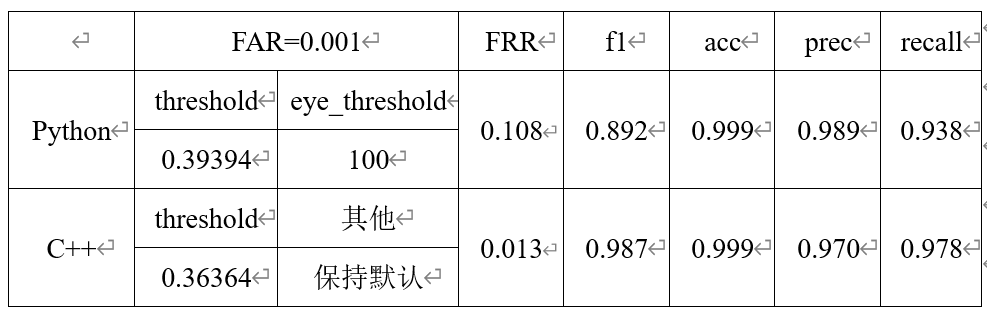展开全文• 基于MATLAB的虹膜识别算法测试平台，很有价值
• 法国库尔布瓦--(美国商业资讯)--在针对虹膜识别性能进行评估的NIST “IREX 10：识别跟踪”(IREX 10：Identification Track)基准测试中，IDEMIA的算法在所有接受评估的公司中高居榜首。 此新闻稿包含多媒体内容。...
法国库尔布瓦--(美国商业资讯)--在针对虹膜识别性能进行评估的NIST “IREX 10：识别跟踪”(IREX 10：Identification Track)基准测试中，IDEMIA的算法在所有接受评估的公司中高居榜首。

增强身份领域的全球领导者IDEMIA证明了虹膜识别的最佳单眼准确性，从而巩固其在生物识别市场中的领先地位。虹膜识别系统使我们能够根据注册证书数据库搜索用户，并在匹配时返回其正确身份。

IDEMIA首席技术官Jean-Christophe Fondeur表示：“IREX 10的结果巩固了IDEMIA自上世纪80年代初以来在生物识别研究领域的领先地位，同时证实IDEMIA团队在该领域的专业知识。在虹膜识别方面排名第一再次彰显我们的技术先进性。过去40年里，我们为在准确性和互操作性方面的显著进步做出了突出贡献，并为之深感自豪。”

就反欺诈和安全性而言，虹膜识别也是最可靠的身份验证解决方案之一。
IDEMIA的技术基于深度学习和人工智能领域的长期专业知识，代表了用于指纹和掌纹识别以及虹膜、人脸和纹身识别的最佳生物识别算法。这些算法在NIST排行榜中一直名列前茅。

什么是NIST IREX 10基准测试？
美国国家标准技术研究所(NIST) 现已划入美国商务部，提供影响广泛的产品和技术同时促进创新和产业竞争力的技术、度量和标准。在此背景下，“IREX 10：识别跟踪”是由NIST提供的开放基准，并由Image Group的生物识别研究实验室管理，它使全世界的开发人员都可以提交各自的匹配软件，以根据虹膜图像库和50万注册用户的算法进行测试。

关于IDEMIA

增强身份领域的全球领导者IDEMIA立志提供值得市民和消费者等信赖的环境，以在物理和数字空间开展重要的日常活动（如支付、联网和旅游）。
确保自身的身份安全已成为我们今天所生活的时代的关键任务。增强身份可确保隐私和信任，并提供安全、经身份验证和可验证的交易保障，通过支持增强身份，我们重塑了我们对于我们最重大的资产之一（身份）的思考、生产、使用和保护方式，不论针对个人还是物体，在安全至关重要的任何时候、任何地方均是如此。我们为金融、电信、身份识别、公共安全和物联网(IoT)领域的国际客户提供增强身份。IDEMIA在全球拥有约13,000名员工，为逾180个国家的客户提供服务。

展开全文• 参考虹膜识别文档： 开源虹膜识别软件OSIRIS4.1的使用入门 将开源虹膜识别算法OSIRIS4.1移植到Windows opencv3.3.0的配置参考(也可以配置其他版本的opencv)： opencv01 相对完整的编译opencv3.3.0 win版本02 ...
01 资源
win10，vs2015，git，opencv3.3.0，cmake
参考虹膜识别文档：开源虹膜识别软件OSIRIS4.1的使用入门将开源虹膜识别算法OSIRIS4.1移植到Windows
opencv3.3.0的配置参考(也可以配置其他版本的opencv)：opencv01 相对完整的编译opencv3.3.0 win版本
02 源码下载
cd D:\git\DeepLearning
git clone --recursive https://github.com/5455945/Iris_Osiris.git
cd Iris_Osiris
# 要记得切换到mfc分支
git checkout mfc

03 配置cmake-gui
源码地址：D:/git/DeepLearning/Iris_Osiris
目标地址：D:/git/DeepLearning/Iris_Osiris/build/x64
设置OpenCV_DIR=C:/Program Files/OpenCV 3.3.0-dirty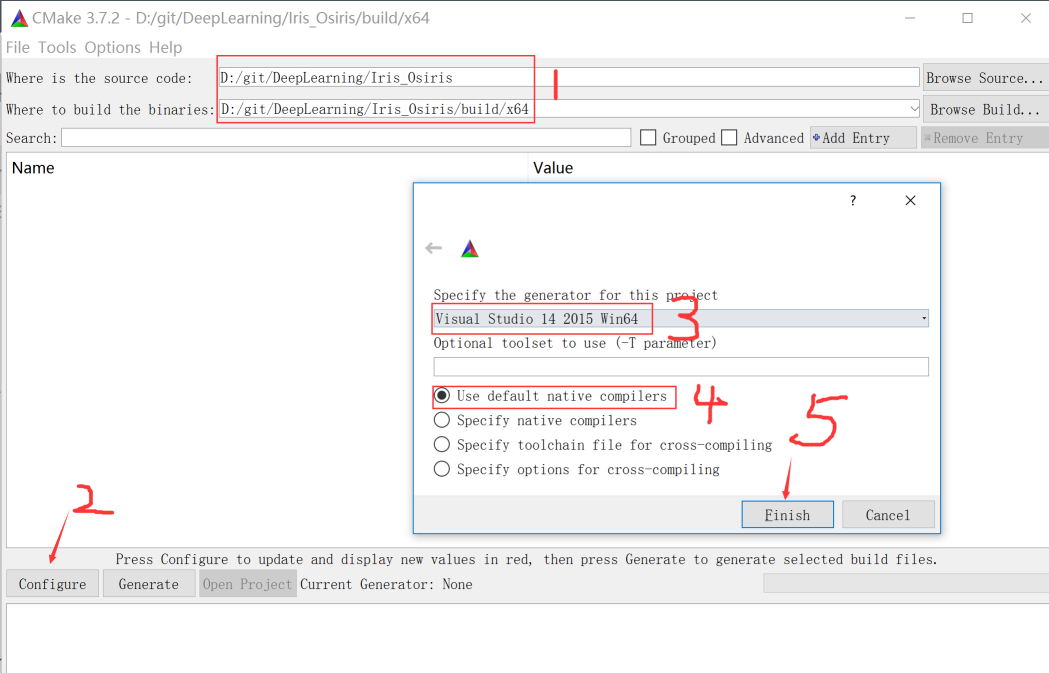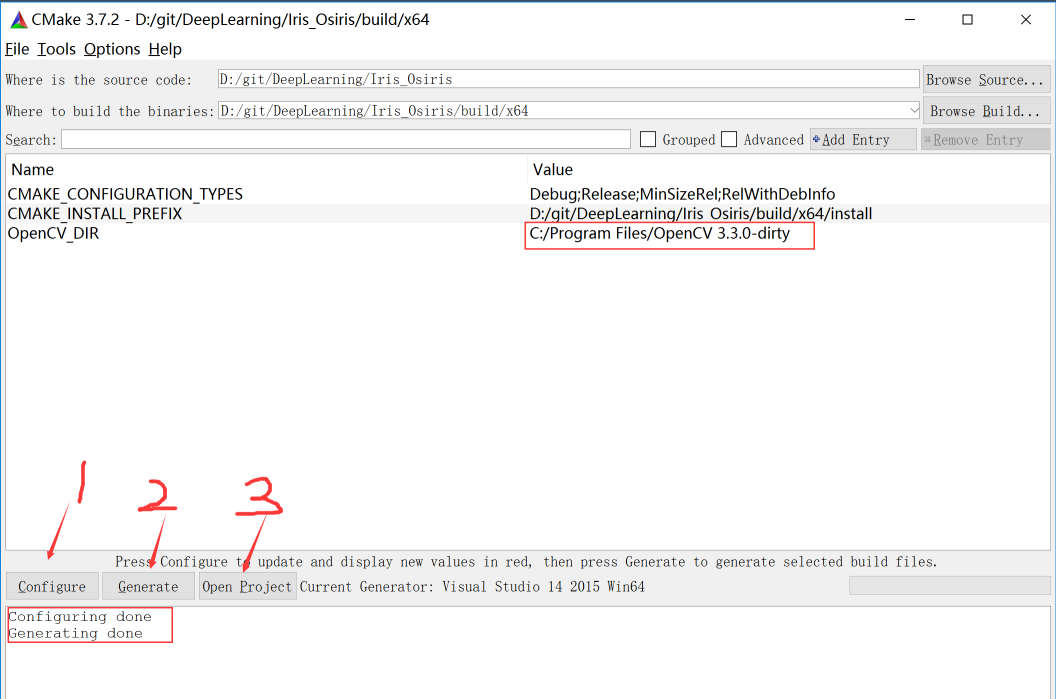04 vs2015编译调试
点击[Open Project]，打开D:\git\DeepLearning\Iris_Osiris\build\x64\Osiris.sln
cmake生成工程文件时，会把需要的input和output目录拷贝到目标位置
D:\git\DeepLearning\Iris_Osiris\build\x64\input
D:\git\DeepLearning\Iris_Osiris\build\x64\output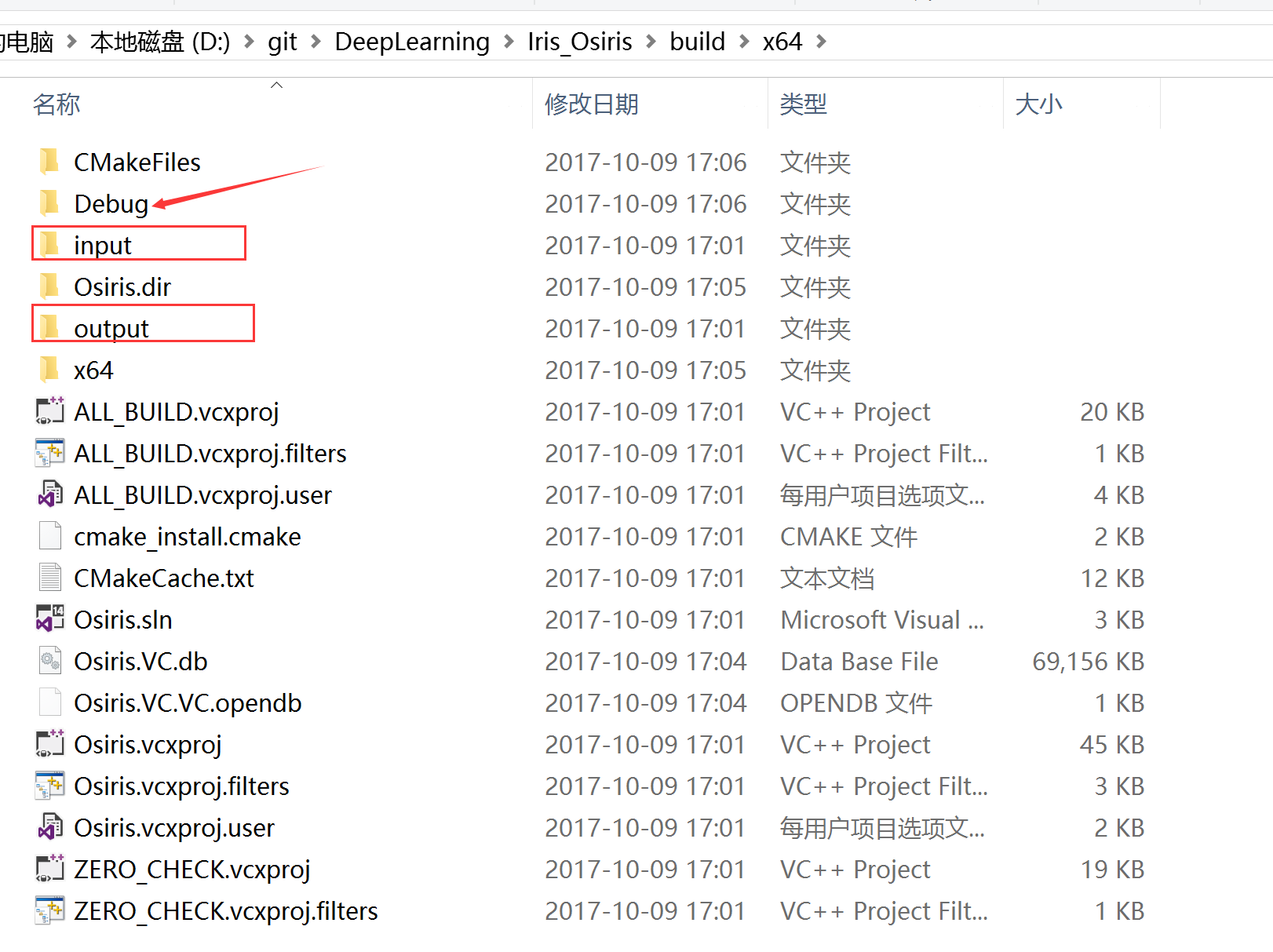F5运行后，如图所示：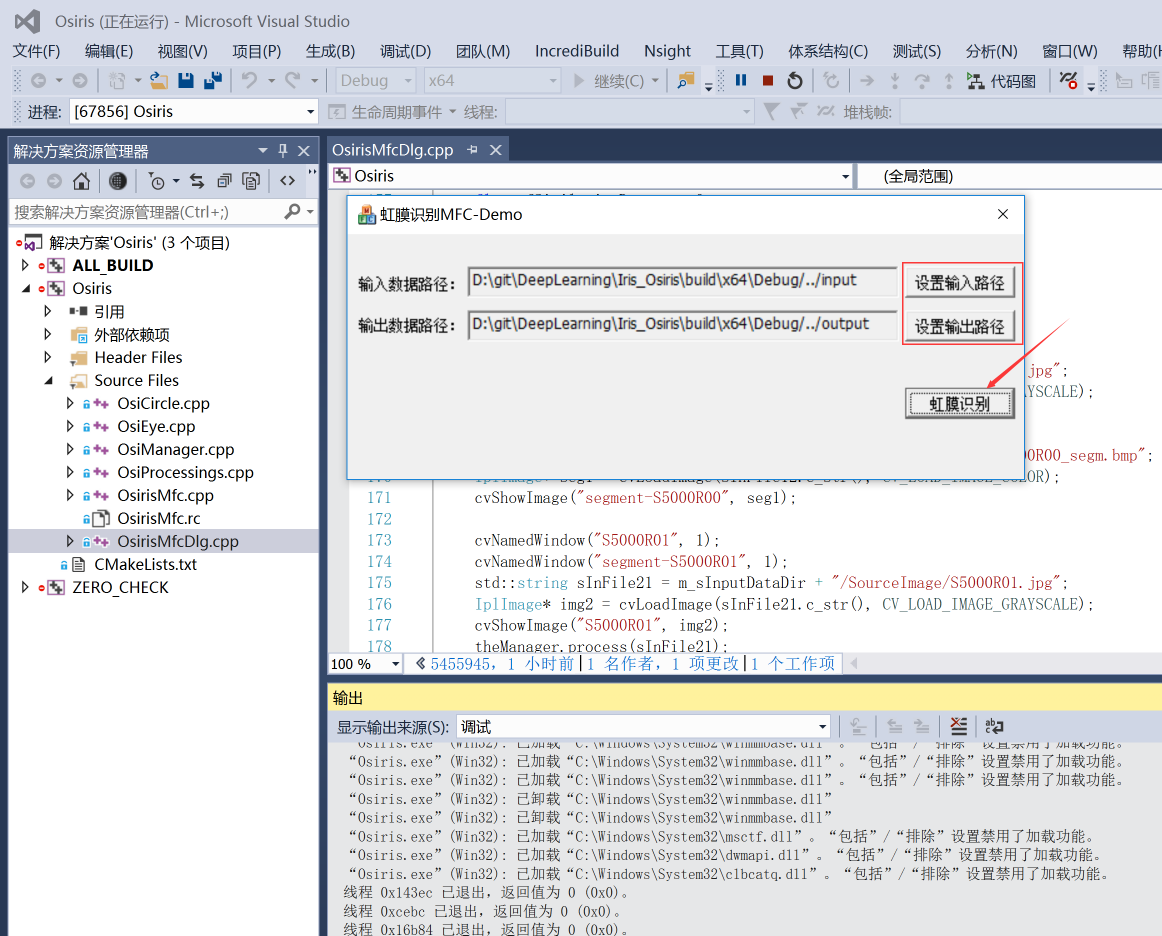点击[虹膜识别]按钮后，效果如下：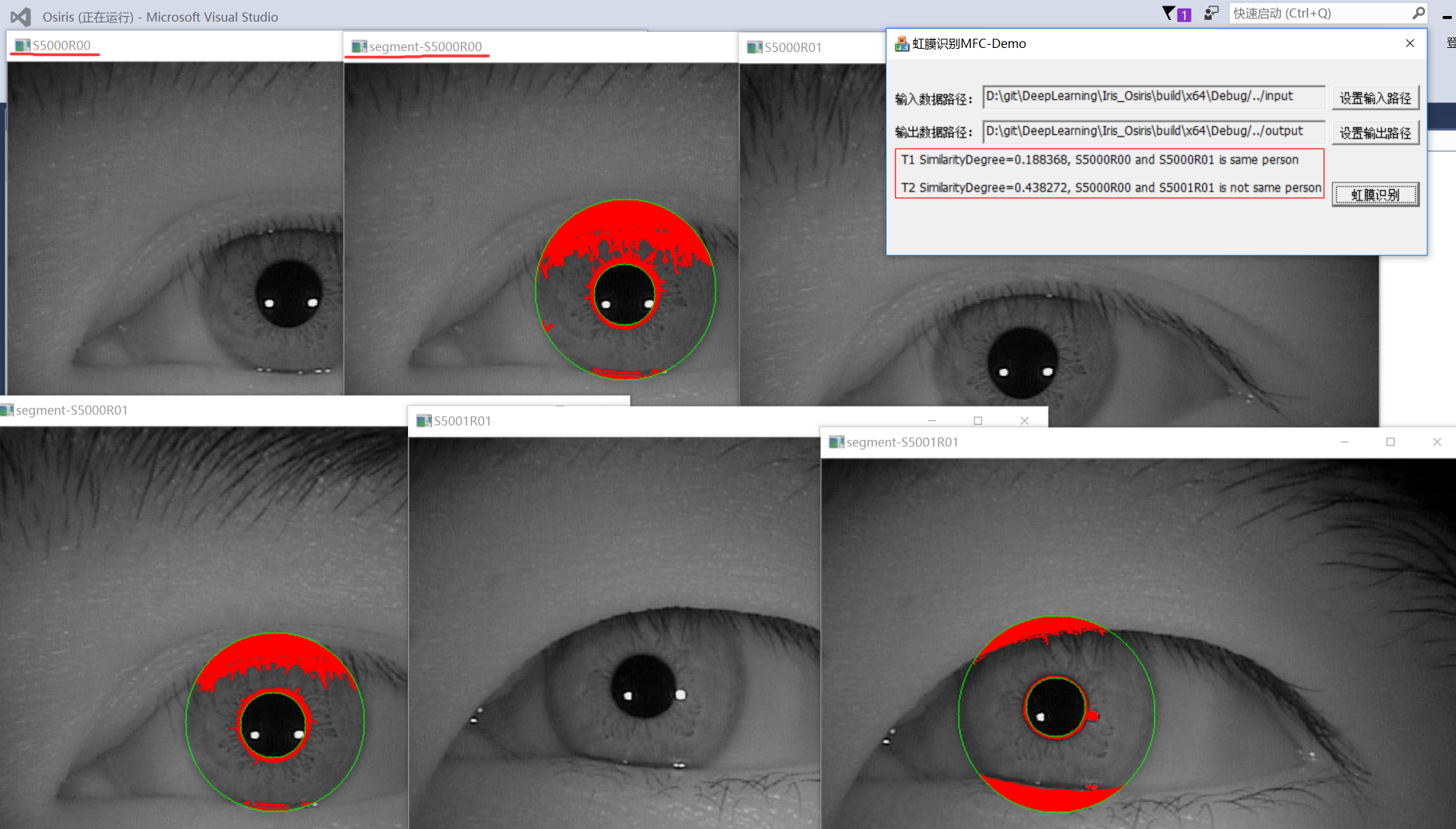展开全文Iris Osiris windows
• 随着图像识别技术的不断发展,人脸识别系统对虹膜识别滤光片的要求不断提高,光在大角度入射时,光谱偏移量往往较大而导致识别失败,因此急需一种低角度...测试结果表明,该膜系结构能满足虹膜识别滤光片的各项技术要求。
• 虹膜识别 项目目标 主要了解虹膜的使用 用于生物识别系统和开发用于提取显着特征的软件用于虹膜扫描的虹膜鉴定。 具体： •识别虹膜的显着特征以及如何使用它们来区分多种 扫描。 •开发用于提取和显示给定...
虹膜识别

项目目标

主要了解虹膜的使用 用于生物识别系统和开发用于提取显着特征的软件用于虹膜扫描的虹膜鉴定。

具体：

•识别虹膜的显着特征以及如何使用它们来区分多种 扫描。

•开发用于提取和显示给定虹膜的显着特征的软件扫描。

•比较虹膜组并检查匹配。

项目范围

该项目一般寻求证明不足和使用虹膜扫描生物识别。鉴定了虹膜的显着特征，分析了它们的提取物批判性地使用软件。开发用于提取特征的软件 MATLAB平台。已经准备好虹膜扫描由于缺乏扫描仪和/或使用 非常高分辨率的相机 否则协助获得所需 用于比较的不同特征。开发的程序将比较两个虹膜图像一段时间，并确定他们是否来自同一个人; 我们是什么指的是匹配。 一种基于Th的计算机视觉算法使用Hough Transform 确定图像中存在的圆圈，其基本上是虹膜和瞳孔。该感兴趣的区域，独特的f获得的食物将位于之间乳头状和边缘状边界。边界是划分虹膜和巩膜的边界。 该比较需要首先通过生成来确定两个虹膜图像的代码他们的单独的生物识别模板然后使用编码程序。虹膜代码之间的距离计算确定它们是否匹配。

文献选取：

1
Libor Masek的MATLAB虹膜识别论文和源代码下载，2003年

http://www.peterkovesi.com/studentprojects/libor/index.html

这个代码很旧了，网上流传也最广，用来入门不错，可以参考作者的论文清晰的看到整个虹膜识别算法的脉络。

而且是用MATLAB写成，在语法上相比C++简洁很多，更加便于理解。当然，前提是，你要很熟悉MATLAB。

2
视频虹膜识别Video-based Automatic System for Iris Recognition (VASIR)源代码
https://www.nist.gov/services-resources/software/video-based-automatic-system-iris-recognition-vasir

基于Qt Framework 4.8.2和OpenCV Library 2.3.1；

可以在多平台上编译运行，最好严格按照指定版本安装qt和opencv，可以用高清摄像头拍一段视频运行试试看。

这个代码很有意思的地方是，他的C++代码是参考上面第一个MATLAB代码改编而来的。

3

《OSIRIS: An open source iris recognition software》2016

http://svnext.it-sudparis.eu/svnview2-eph/ref_syst/Iris_Osiris_v4.1/

目前效果最好的开源虹膜识别源代码，基于C++和OpenCV，该链接中包含使用文档和源代码，

论文下载链接：http://www.docin.com/p-1389745651.html

这个源码非常优秀，效果很好，已经是4.1版了。用它改编成一个商业应用的虹膜识别软件也没那么

难。

人类虹膜

虹膜位于人眼中在角膜和晶状体之间的区域。它控制着进入眼睛的光量 瞳孔。这是通过使用它它所特有的专用肌肉称为括约肌和扩张器。它是一种可见内脏器官来自外部，重要的是要注意它从出生到死亡一直保持不变形成了一个合适的生物识别工具。 形成虹膜表面的独特图案在生命的第一年。独一无二的图案是随机形成的与遗传因素无关。这种表观遗传性质解释为什么同一个人的左右虹膜永远不会相似。他们的虹膜图案也会有所不同。虹膜识别技术是合适的，因为即使是同卵双胞胎也不能拥有同的虹膜 模式。个体的左眼和右眼也具有不同的虹膜纹理图案。它是从外面可见的唯一内部器官，并且在年轻之后也没有太变化。

虹膜识别的优缺点

优点

假阳性发生率低。
非常低（几乎0％）的假阴性测试。
高度可靠的双胞胎虹膜图案不同。
快速的结果：非常快速地验证了主题的身份。
虹膜从出生到死亡保持不变，因此适用于广泛的应用。

缺点

测量的准确性可能会受到白内障等疾病的影响散光。
扫描程序被一些人认为是侵入性的。
设备成本高。

图像分割

解释了这里使用的技术。 霍夫变换 这是一个标准的计算机视觉算法用于确定参数 简单的几何对象，如线条 和图像中的圆圈。人们可以使用圆形Hough变换确定e中心和半径虹膜和瞳孔 区域。您首先生成边缘map通过计算的一阶导数 眼睛图像中的强度值，然后对结果进行阈值处理。从边缘地图，投票在霍夫空间投下 圆的参数通过每个边缘点。这些参数是中心坐标和半径圈。他们将使用通常的等式定义任何圆。

encode编码

这需要编码虹膜图像纹理到一个矢量代码。 在大多数算法中，过滤器用于获取信息虹膜纹理。然后输出滤波器被编码为位向量代码。Gabor和log-Gabor滤波器是例子这样可以用于该过程。对数例如，Gabor过滤器被引入 提取和规范化的特征。它将提供将被量化的相位数据用于生成逐位生物识别模板。只有最具辨别力的特征使用虹膜。匹配阶段计算距离两组虹膜代码和决定 是否匹配（在验证上下文中）或识别提交的探测虹膜 来自画廊集中的主题。（识别阳离子背景）。Calcula比率称为汉明距离是必不可少的，因为它是适当的选择比较两个虹膜和 确定他们是否来自同一个人（匹配）。 虽然使用了许多算法 虹膜识别（例如：Boles，Boas hash，Wildes，Daugman），常见的是Daugman和Wild的计划 实现。

Daugman技术

Daugman算法是最着名的虹膜识别算法。虹膜是建模的作为两个不一定同心的圆。每个圆圈由三个定义参数（x该，和该，r），其中（x该，和该）找到半径为r的圆心。它利用积分微分算子，用于估计每个圆的三个参数值 边界。它相对于增加的半径r搜索整个图像以最大化

步骤

输入光圈

只需接受已扫描的虹膜图像进行比较。由于缺乏数据采集机器，图像来自中国科学院C Hinese A cademyof S ciences(www.sinobiometrics.com)庞大的数据集。该网站是能够提供一些 已经清晰扫描过的虹膜图像接近。这确保了缺席反射因此消除了计算错误。

虹膜本地化

本地化过程由不同模块中的许多算法执行。我们有一个是圆形发生器，它将权重加到霍夫累加器阵列中。 霍夫变换用于在图像中找到圆圈。虹膜图像的边缘经历了精确的边缘检测方法。然后开发一个模块用于返回通过使用霍夫变换和canny边缘在图像中的圆的坐标 检测以创建边缘图像。 线和圆坐标被确定用于返回a的像素坐标 由给定半径和x，y坐标定义的圆。图像伽玛调整是执行以增强亮区的对比度（对于图像伽马值范围0-1）和 还可以增强暗区的对比度（值> 1）。 执行图像的滞后阈值处理。这里，所有像素的值都高于a给定的预设阈值标记为边缘。用于实际虹膜分割的模块也用于消除噪音，例如遮挡眼睑和睫毛引起的噪音消除 也包括在内。

虹膜归一化和编码

虹膜图像的标准化是有效的通过将圆形区域展开成一个 矩形块常数 尺寸。规模的影响虹膜图像中的参考可以 要明显并防止 我们在此之前进行标准化 编码。规范化有帮助补偿虹膜变形。我们解开了e图像到固定的矩形块 尺寸。然后通过使眼睑变暗来遮盖眼睑区域。使用虹膜图像从笛卡尔坐标转移到极坐标图像坐标Daugman橡胶板模型如公式3.1和图3.3所示。通过使用1D Log-Gabor滤波器对图像的每一行进行卷积生物识别模板然后从标准化生成虹膜图像。我们将说明如何编码给出虹膜图像行。编码将属于将虹膜的位矢量代码用于匹配阶段的比较。Gabor滤波器的输出具有实部和虚部。经过分解后1D log Gabor滤波器，每个输出的相位角量化为2位。

汉明距离（匹配）

这确定虹膜图像是否匹配。小汉明之间的距离代码表示更好的匹配。汉明距离（HD）定义为两个代码中不同位的数量除以有效位的总数。

工学硕士学位论文：虹膜病理特征提取研究

Classified Index: TP391.4 U.D.C: 004.9

《Dissertation for the Master Degree of Engineering RESEARCH ON IRIS PATHOLOGICAL FEATURE EXTRACTION 》

现在国内对虹膜诊断系统的研究还比较少。目前，主要是哈尔滨工业大学计算机系生物信息技术研究中心致力于计算机辅助虹膜诊断的研究。另外，原华中理工大学机械科学与工程学院的一个研究小组曾发表过关于虹膜诊断系统的论文，他们提出了多任务虹膜诊断专家系统的概念，并给出了一个抽象的系统模型：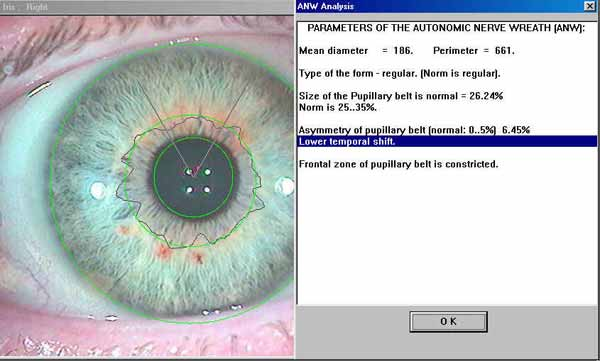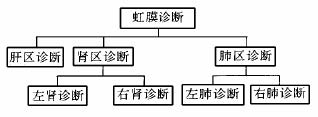图 1-4 文献中的虹膜诊断系统模型   Figure 1-4 Iris diagnosis system model in 

虹膜诊断学(Iridology)己经经历了一百多年的发展，逐渐形成了一套完整的体系。虹膜诊断是利用个体虹膜图像中发生变异的细节结构，如虹膜中出现的坑洞、裂缝、斑块、线条、颜色变化，通过分析虹膜的这些异常达到疾病诊断的目的。因此，研究基于计算机软件的虹膜病理特征提取是实现计算机辅助虹膜诊断的基础。

从虹膜图像的结构纹理特征入手，对虹膜图像的病理特征进行提取。主要研究内容包括以下几个方面：
(1) 改进虹膜识别中的虹膜定位方法。首先利用眼睛图像的灰度分布粗略定位瞳孔，再通过变形模板的方法精确定位虹膜内外边缘。该算法很好地适应了当前采集设备所采集的虹膜图像，使定位精度达到像素级。

(2) 虹膜七环是分隔人体几大代谢功能的重要分界线，尤其是虹膜自主神经环，在虹膜诊断中有着重要的作用。本文研究了基于Snake 模型的自主神经环提取方法，对传统的轮廓搜索方法提出了改进，避免了轮廓收敛到局部能量最小值，从而检测到真实的自主神经环。

(3) 本文针对虹膜学中定义的线条、裂缝、坑洞三种病理特征进行了提取。采用基于Canny 边缘检测的方法对虹膜的线条病理特征和坑洞病理特征进行提取，并提出基于高斯滤波的裂缝病理特征检测方法。

(4) 虹膜学把虹膜划分成与身体各个器官对应的小区域，本文根据图谱对虹膜的划分，把虹膜图谱精确地影射到真实的虹膜图像上，从而实现虹膜的自动图谱覆盖，并可根据鼠标的位置报告虹膜分区的名称。

虹膜识别可以应用于人体健康状态的检测。虹膜学是一门以形态学为 基础，可以透过眼睛虹膜的变化来推断个人健康状况及窥视康复过程的学科。

现代医学研 究发现眼睛的虹膜是人脑的延伸，赋予千千万万神经末梢、细微的血管、肌肉及其它组织， 因此虹膜上的神经纤维可见展示反射生理学，而对身体健康的变化做出相应的反应。

话不多说，先上一个关于虹膜左右眼反射区域的对照表：https://wenku.baidu.com/view/2afb5a40f121dd36a22d82da.html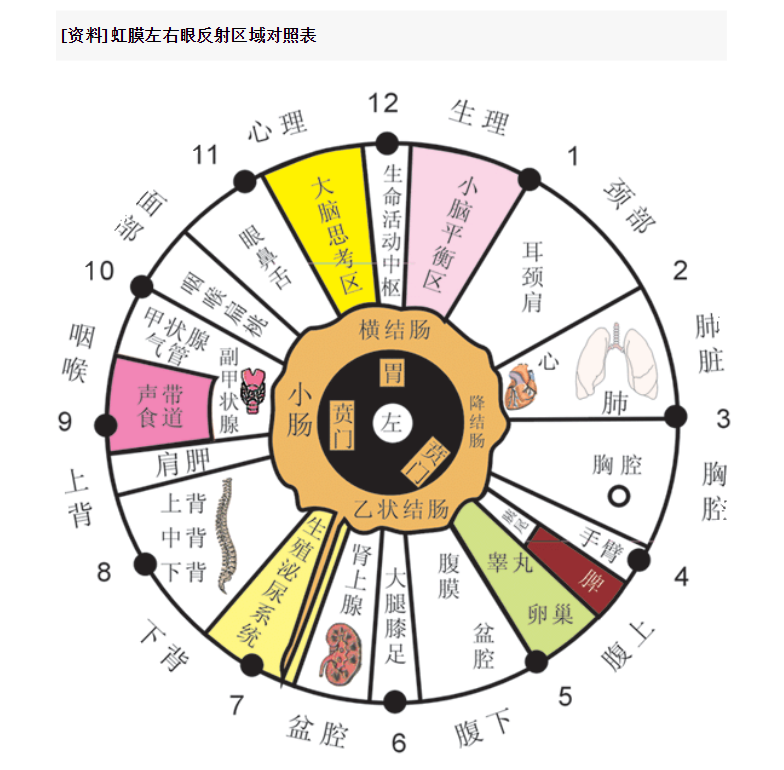这里取几例器官与虹膜相关的六大现象分类：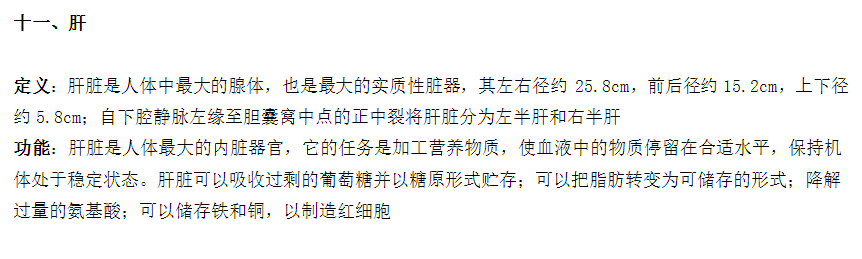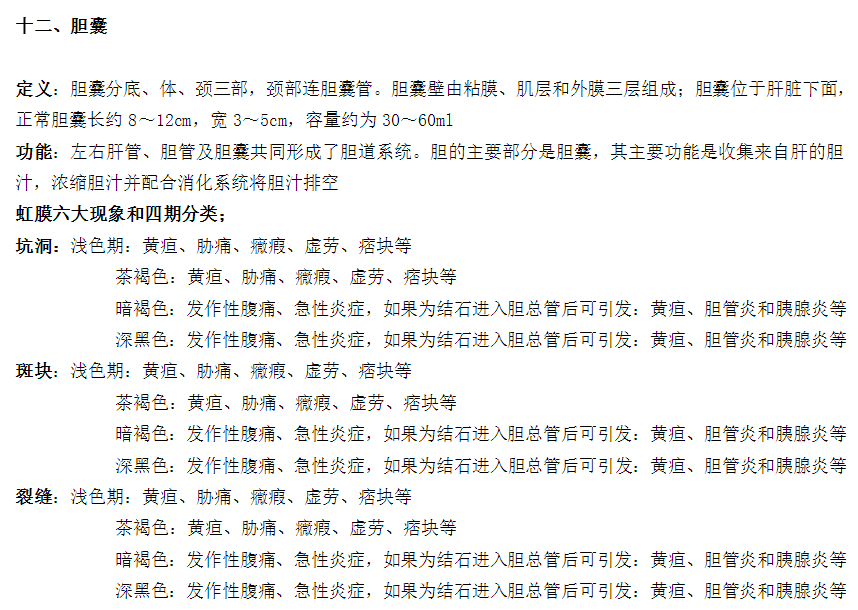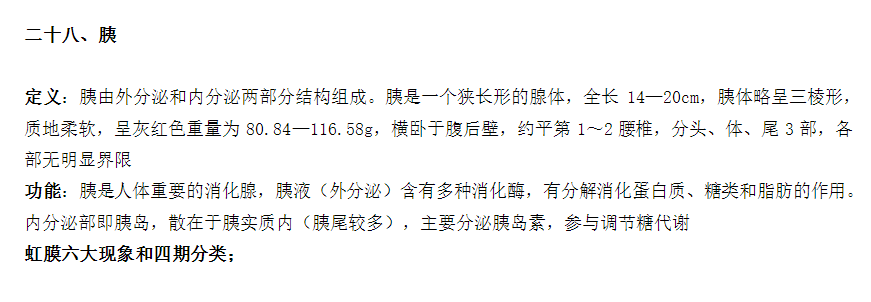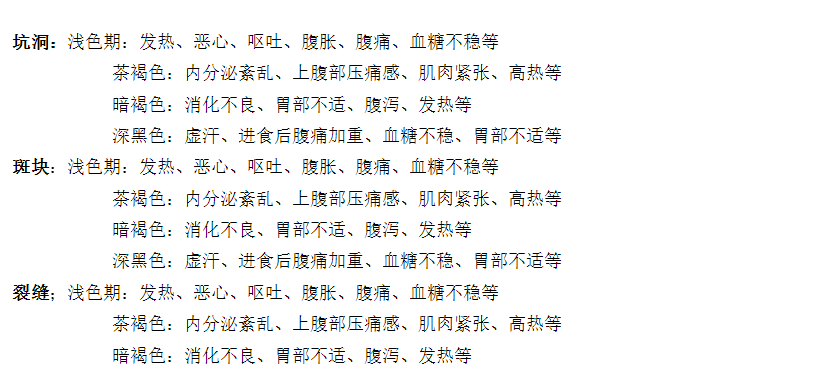虹膜左右眼的反射图：

https://wenku.baidu.com/view/6241ea4db307e87101f6966d.html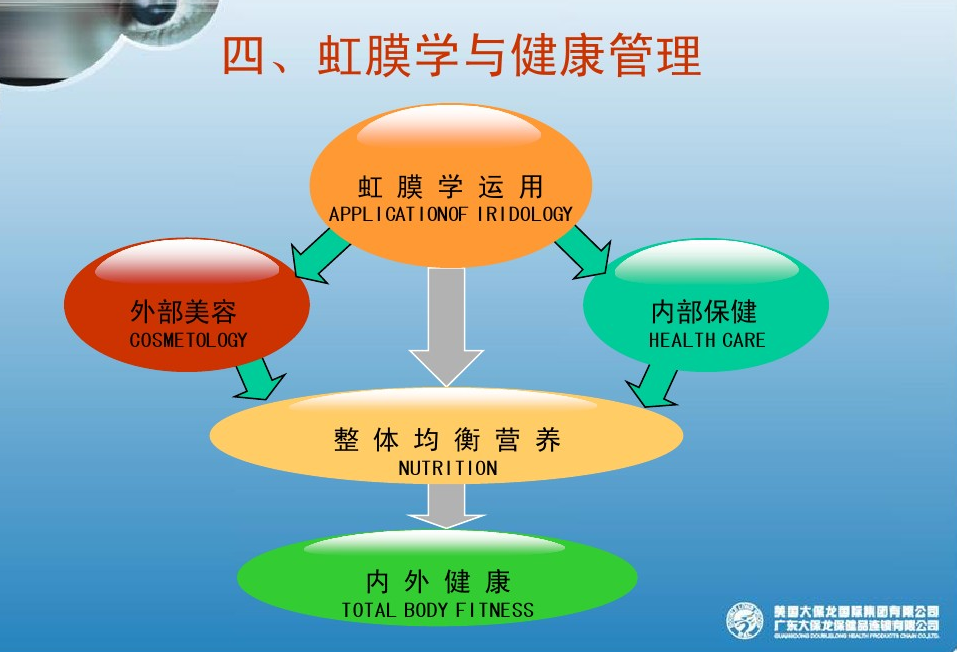《虹膜诊断学--让眼睛说话》2012-国际版正式发行!

https://wenku.baidu.com/view/3c69900976c66137ee0619a5.html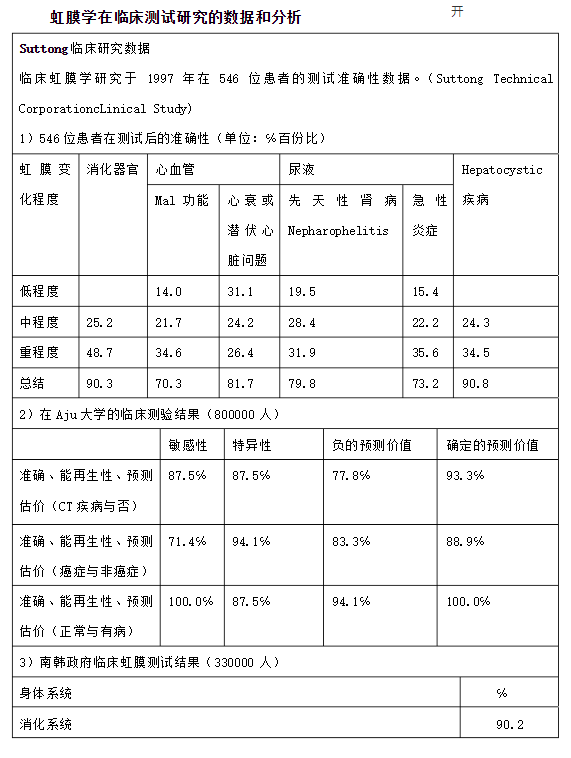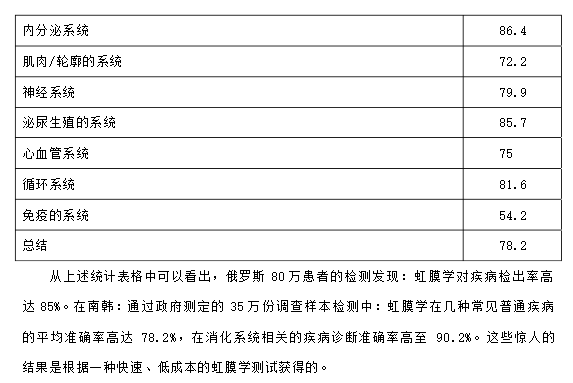望眼诊病.pdf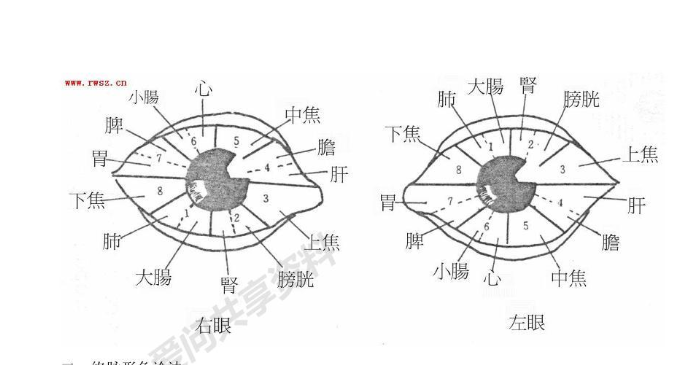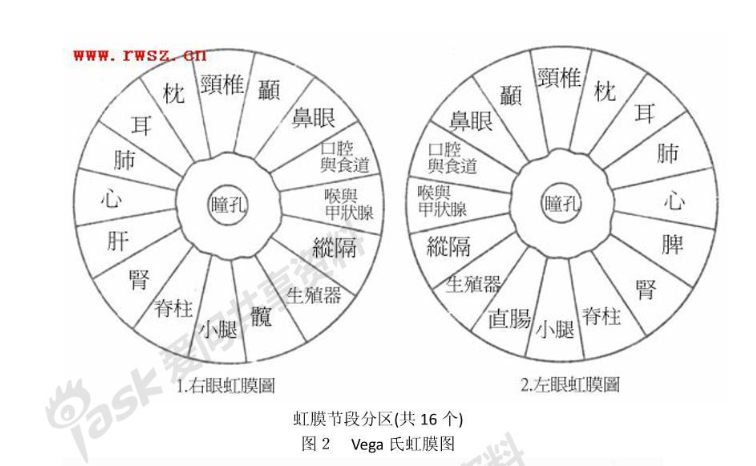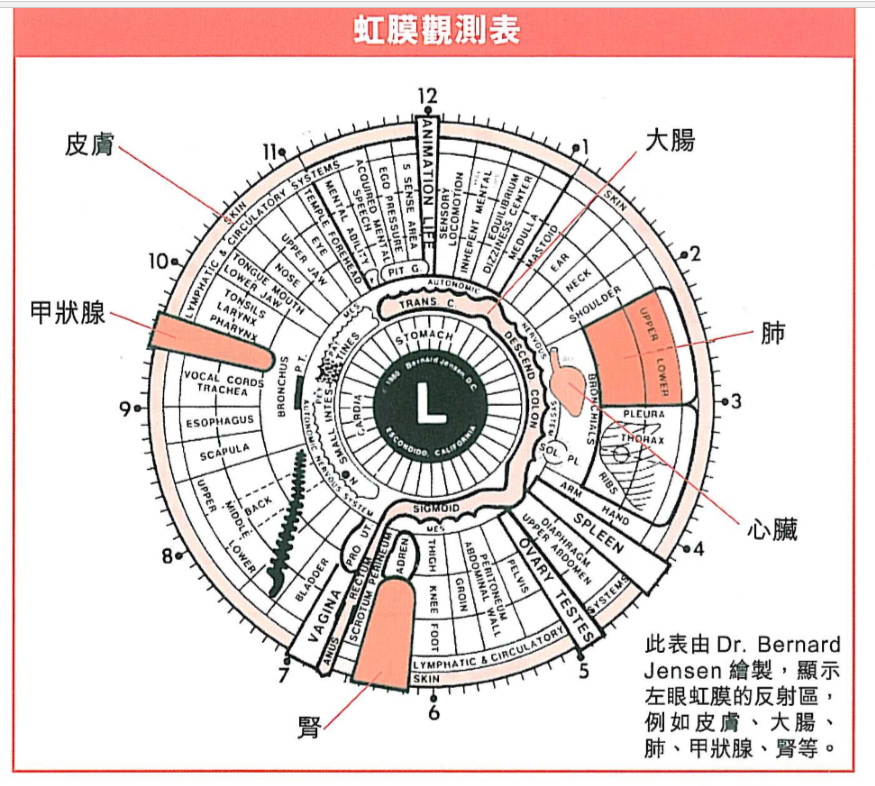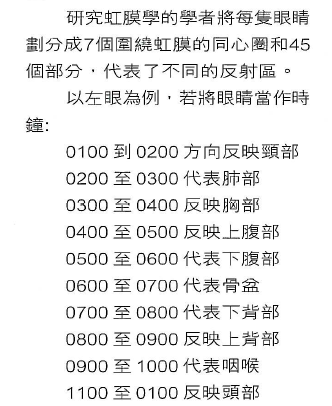展开全文特征点
• 一个详尽的虹膜识别matlab源代码，采用霍夫变换的方法进行图像分割，利用海明距离进行判别，内附有虹膜库一个（6个库图像），三张虹膜测试图像
• 在http://blog.csdn.net/piaoxuezhong/article/details/77966132中大致总结的虹膜识别与虹膜定位的原理，本篇结合现有的方法，实例测试实现，做下记录： 将开源虹膜识别算法OSIRIS4.1移植到Windows： ...
• 为了提高虹膜识别的准确率，提出了一种改进曲波变换的虹膜识别算法。首先对预处理后的虹膜图像进行Wrapping算法的快速离散曲波变换，提取不同尺度和不同方向的曲波子带系数矩阵的均值、方差和能量，然后利用广义高斯...广义高斯分布 曲波变换 模糊支持向量机 二叉决策树
• Learning Deep-IrisCodes from Scratch for Segmentation-Robust Visible Wavelength and Near Infrared Iris Recognition，《从零开始学习深层虹膜图像分割鲁棒可见光和近红外虹膜识别》，一篇关于虹膜识别的论文...神经网络
• GIRIST (GRUS IRIS TOOL) 是最新的虹膜识别软件，其性能与商业软件相当. 发布于http://www.grusoft.com/girist.htm。 GIRIST特性如下： 平均Decidability > 6.0 平均识别率>95% FAR=0.01%, 平均 FRR=5% ...
• 针对现有二维码安全技术的不足，设计并实现了基于WPKI和虹膜识别的二维码安全管理系统。该系统通过WPKI技术，利用数字证书对杂凑处理后的信息进行签名，供用户对二维码的来源进行可信性验证；通过建立公安户籍管理...二维码 数字签名
• 中国科学院自动化研究所模式识别国家重点实验室研究员、天津中科智能识别产业技术研究院院长孙哲南研究员就虹膜识别这一话题做了详细的讲解。 今年是人工智能60周年，像虹膜、人脸、指纹识别是比较实用的人工智能，...
• hello，这是鑫鑫鑫的论文分享站，今天分享的文章是An Experimental Study of Deep Convolutional Features For Iris Recognit ，一篇关于虹膜识别的论文，一起来看看吧~ 摘要： 虹膜识别大多数都是基于生物识别专家...神经网络
• 传统的虹膜识别系统需要将虹膜图像转换至极坐标系统并进行归一化, 通过平移特征向量来达到旋转不变性。为了降低传统虹膜识别方法的复杂性, 提出了一种融合局部与全局特征提取的虹膜识别方法, 无须对预处理后的虹膜...
• 中科院虹膜数据库，可以用于做虹膜识别，提取等等。非常全面，我们做项目也在用。大量测试的时候很好用。
• hello，这是鑫鑫鑫的论文分享站，今天分享的文章是Iris Recognition With Off-the-Shelf CNN Features: A Deep Learning Perspective ，一篇关于虹膜识别的论文，一起来看看吧~ 摘要： 虹膜识别是指根据虹膜特征...神经网络 机器学习 pytorch
• 虹膜是可靠的人类识别的强大工具。... 提出的虹膜识别系统在四个公共数据集 IITD、虹膜数据库 CASIAIris-V1、CASIA-Iris-thousand 和 CASIA-Iris-V3 Interval 上进行了测试。 该系统以非常高的准确率取得了优异的结果。
• 为验证算法性能，采用MICHE I虹膜图库中由iPhone5拍摄所得的30人240张（每人4张室内和4张室外）虹膜图像进行测试，并与Gabor变换和主成分分析虹膜识别方法进行对比。结果表明，该方法在室内外图像均进行训练的条件下...
• 为提高虹膜识别的准确度,提出采用第二代曲波变换提取虹膜图像纹理特征的方法。首先将预处理后的虹膜图像进行曲波分解,然后采取不同方法对曲波分解后的低通和带通子带进行特征提取,并根据各带通子带的能量大小赋予...
• hello，这是鑫鑫鑫的论文分享站，今天分享的文章是DeepIrisNet: DEEP IRIS REPRESENTATION WITH APPLICATIONS IN IRIS RECOGNITION AND CROSS-SENSOR IRIS RECOGNITION ，一篇关于虹膜识别的论文，一起来看看吧~ ...神经网络
• 为了提高虹膜识别系统的识别性能，针对虹膜识别中的特征提取与模式分类问题，提出了一种基于核Fisher鉴别分析(kernel fisher discriminant analysis，KFDA)与支持向量机(support vector machine，SVM)的虹膜识别方法...
• 深度虹膜表示在虹膜识别和交叉传感器虹膜识别中的应用 Abhishek Gangwar 2016年 ICIP ABSTRACT 尽管虹膜识别技术取得了重大进展，但大规模和非理想条件下的高效和鲁棒的虹膜识别仍然存在严重的性能问题，仍是当前的...
• 为了解决传统虹膜识别系统在非理想虹膜图像下识别性能不够好的问题，提出了基于纹理方向能量特征的虹膜识别方法。该方法先设计一组水平与垂直的方向滤波器提取虹膜的纹理边缘，比较虹膜纹理边缘在两个方向的能量强度...
• 自从今年8月三星Note7发布带有虹膜识别功能后，陆陆续续有新闻爆料华为Mate9、Iphone8等也将都带有虹膜识别功能，虽然华为Mate9发布后否定了这个信息，但从侧面可以看出虹膜识别技术现在所受关注度非常高，也必定是...
• matlab眼睛识别源码虹膜识别 这是基于 . 可以找到他在 MatLab 和 Python 中实现的原始虹膜识别系统。 描述 该系统对于实际应用程序来说是不完整的。 一个完整的系统必须有一个特定的相机来捕捉眼睛的虹膜。 然而，...
• 概要本文会详细的介绍如何改装苹果手机，使其具有虹膜识别的功能二．目标、成果使用苹果手机实现虹膜的注册、登录、匹配识别的功能，在苹果4s可以进行真机验证、在苹果6s上的速度&lt;0.05s三．改装简介1. 硬件a...生物识别
• https://blog.csdn.net/piaoxuezhong/article/details/78503740 在http://blog.csdn.net/piaoxuezhong/article/details/77966132中大致总结的虹膜识别与虹膜定位的原理，本篇结合现有的方法，实例测试实现，做下...
• 这个基于MATLAB的框架允许自动评估识别过程的所有四个阶段（分段，归一化，编码和匹配）中的虹膜识别算法，并将其与执行相同功能的其他算法互换。 可以从可用算法的列表中选择每个阶段的算法，并且对于子功能也可以...开源软件...